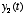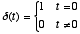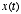NPB 163/PSC 128

Linear time-invariant systems and convolution

Linear, time-invariant systems

• Let us consider a dynamical system with inputand output:• Such a system is said to be a linear, time-invariant system if it obeys the laws of superposition and scaling over time. That is, if you observe an output signalin response to an input signal, and you later observe an outputin response to input, then the response to the combinationis just.

• One way to characterize a linear, time-invariant system is by measuring its impulse response function. This is the response you would obtain to a small pulse of unit amplitude,, whereLet’s call the output signal we measure in response to such an input.

• Once we have measured the impulse response function,, we can compute the outputin response to any complex input. The first step is to consider the signalto be composed of a superposition of unit impulses of different amplitudes:Now since the system is also time-invariant (i.e., it does not change its behavior over time), then we know that the response to a shifted impulseis just. And so the response to a weighted sum of such shifted impulses is just a weighted sum of the resulting shifted impulse response functions. Or in the language of mathematics:• Thus, the outputin response to the input signalmay be written as(1)

And in the limit that the spacing between time samples becomes infinitesimally small, this relation becomes exact and the sum turns into an integral:(2)

Convolution
• The expression above is known as the convolution sum (1) or convolution integral (2). It tells us how to predict the output of a linear, time-invariant system in response to any arbitrary input signal.

• The mathematical shorthand notation for the convolution operation is to use thesymbol as follows:• One way of interpreting the convolution sum is just as we developed it above - i.e., it is simply a linear superposition of impulse response functionseach of which is multiplied by.

• The other (more common way) of interpreting the convolution sum is that it tells us that the output is computed by taking a weighted sum of the present and past input values. We can see this by writing out the sum in (1) above:where we have assumed here for now that the timesare spaced by one unit of time. Note also that we do not sum over values offor which. The reason is that for any physical system,is defined only for. This indeed makes sense, because otherwise we would need to know future values of the input in order to compute the present output.

• The convolution operation may also be thought of as a filtering operation on the signal, where the impulse response functionis acting as the filter. The shape ofdetermines which properties of the original signalare “filtered out.” The design of filters is usually best thought of in the frequency domain, which we turn to next....